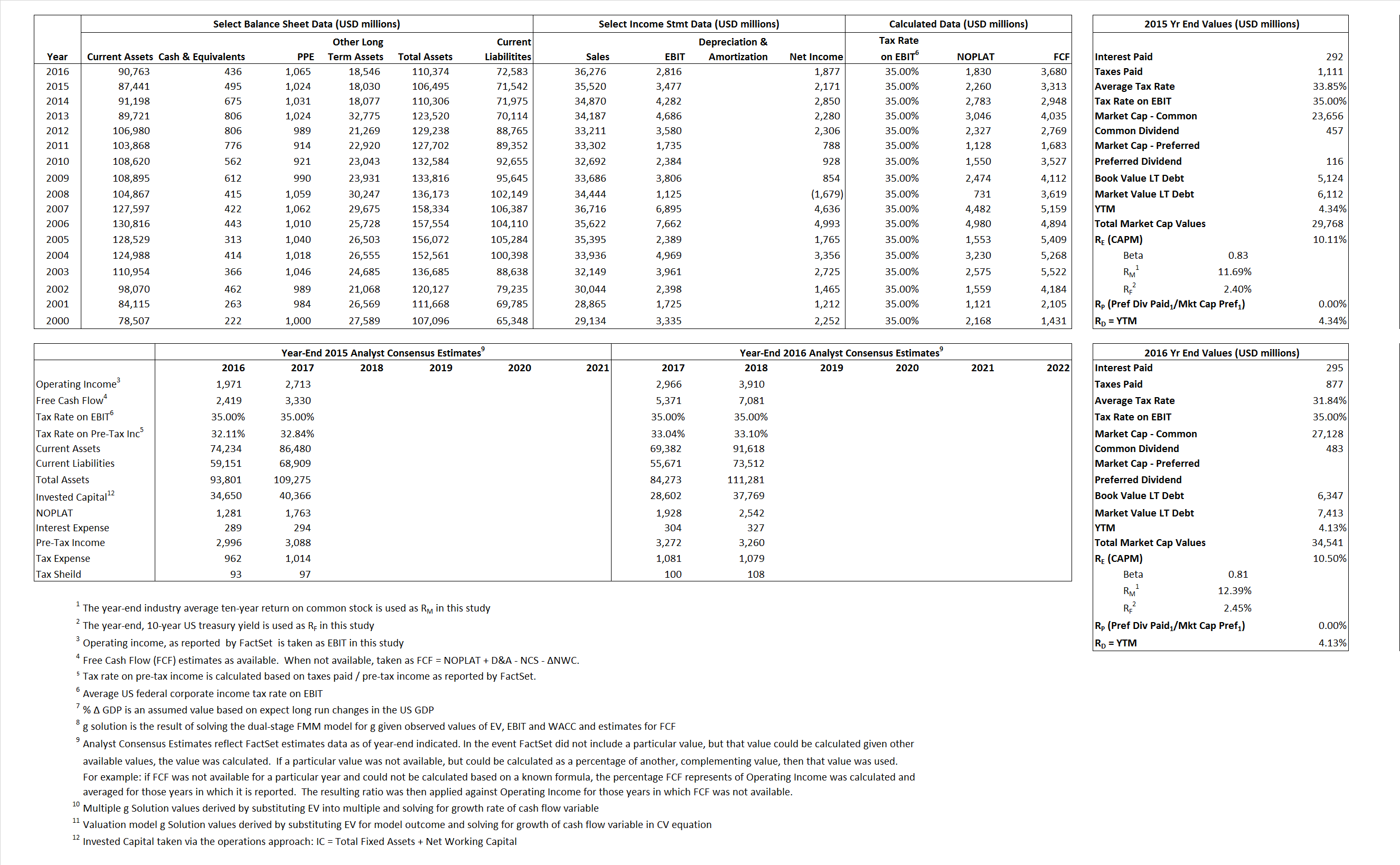# Allstate

## Analyst Listing

The following analysts provide coverage for the subject firm as of May 2016:

 Broker Analyst Analyst Email William Blair Adam Klauber aklauber@williamblair.com Morningstar Brett Horn brett.horn@morningstar.com Raymond James C. Gregory Peters greg.peters@raymondjames.com Piper Jaffray Daniel D. Farrell daniel.d.farrell@pjc.com Wells Fargo Securities Elyse Greenspan elyse.greenspan@wellsfargo.com Dowling & Partners Gary Ransom ransom@dowling.com Argus Research Jacob Kilstein jkilstein@argusresearch.com Bernstein Research Josh Stirling josh.stirling@bernstein.com Deutsche Bank Research Joshua Shanker joshua.shanker@db.com Compass Point Research Ken Billingsley kbillingsley@compasspointllc.com RBC Capital Markets Mark Dwelle mark.dwelle@rbccm.com JMP Securities Matthew J. Carletti mcarletti@jmpsecurities.com Keefe Bruyette & Woods Meyer Shields mshields@kbw.com Sandler O’Neill & Partners Paul Newsome pnewsome@sandleroneill.com FBR Capital Markets & Co Randy Binner rbinner@fbr.com Credit Suisse Ryan Tunis ryan.tunis@credit-suisse.com Sterne Agee CRT Vinay Misquith vmisquith@sterneageecrt.com

## Primary Input Data## Derived Input Data

### Equational Form

Net Operating Profit Less Adjusted Taxes NOPLAT 2,260 1,830$NOPLAT\, =\, EBIT\, x\, (1 \,-\, Avg \,\,Tax\,\, Rate\,\, on\,\, EBIT)$
Free Cash Flow FCF 3,313 3,680$FCF\,=NOPLAT\,+\,Non-Cash\,Expenses-\Delta NWC\,-\,NCS$
Tax Shield TS 99 94$TS\,=\,Interest\,\,Paid\,\,x\,\, Avg \,\,Tax\,\,Rate\,\, on\,\, Pre-Tax\,\, Income$
Invested Capital IC 34,953 37,791$IC\,=\,Fixed\,\,Operating\,\,Assets\,\,+\,\,Net\,\, Working\,\, Capital$
Return on Invested Capital ROIC 6.47% 4.84%$ROIC\,=\,\frac { NOPLAT }{ IC }$
Net Investment NetInv (3,378) 2,838$NetInv\,=\,{ {IC}_{1}}-{{IC}_{0}}+Depreciation$
Investment Rate IR -149.47% 155.05%$IR\,=\,\frac {NetInv}{NOPLAT}$
Weighted Average Cost of Capital
WACCMarket 8.62% 8.85%$WACC\,=\,\frac { E }{ V } { R }_{ E }\,+\,\frac { P }{ V } { R }_{ P }\,+\,\frac { D }{ V } { R }_{ D }\left( 1- Avg\,\, Tax\,\,Rate\,\,on\,\,Pre-Tax\,\,Income \right)$
WACCBook  7.05%  6.70%
Enterprise value
EVMarket 29,273 34,105$EV\,=\,Market\,\,Cap\,\,Equity\,+\,\,Long\,\,Term\,\,Debt\,-\,Cash$
EVBook  28,285  33,039
Long-Run Growth
g = IR x ROIC
-9.66%  7.51% Long-run growth rates of the income variable are used in the Continuing Value portion of the valuation models.
g = %$\Delta$ GDP  2.50%  2.50%
Margin from Operations M 9.79%  7.76%$M\,\,=\,\,\frac{EBIT}{SALES}$
Depreciation/Amortization Rate D 0.00%  0.00%$D\,\,=\,\,\frac{D+A}{EBITDA}$

## Valuation Multiple Outcomes

The outcomes presented in this study are the result of original input data, derived data, and synthesized inputs.

### model g solution

12/31/2015 12/31/2016 12/31/2015 12/31/2016 12/31/2015 12/31/2016

EV/SALES$\frac {EV}{Sales} \,= \,\frac{ROIC\, -\, g}{ROIC\,(WACC\,-\,g)}\,(1\,-\,T)\,(M)$

.82  .94  -4.66%  -32.24%  -3.22%  66.69%

EV/EBITDA$\frac {EV}{EBITDA} \,= \,\frac{ROIC\, -\, g}{ROIC\,(WACC\,-\,g)}\,(1\,-\,T)\,(1\,-\,D)$

8.42 12.11 -4.66% -32.24% -3.22% 66.69%

EV/NOPLAT$\frac {EV}{NOPLAT} \,= \,\frac{ROIC\, -\, g}{ROIC\,(WACC\,-\,g)}$

12.95 18.63 -4.66% -32.24% -3.22% 66.69%

EV/FCFOPS$\frac {EV}{FCF_{OPS}} \,= \,\frac{ROIC\, -\, g}{ROIC\,(WACC\,-\,g)}\,(1\,-\,T)$

8.84 9.27 -4.66% -32.24% -3.22% 66.69%

EV/EBIT$\frac {EV}{EBIT} \,= \,\frac{ROIC\, -\, g}{ROIC\,(WACC\,-\,g)}\,(1\,-\,T)$

8.42 12.11 -4.66% -32.24% -3.22% 66.69%

EV/IC$\frac {EV}{IC} \,= \,\frac{ROIC\, -\, g}{WACC\,-\,g}$

.84 .90 -4.66% -32.24% -3.22% 66.69%